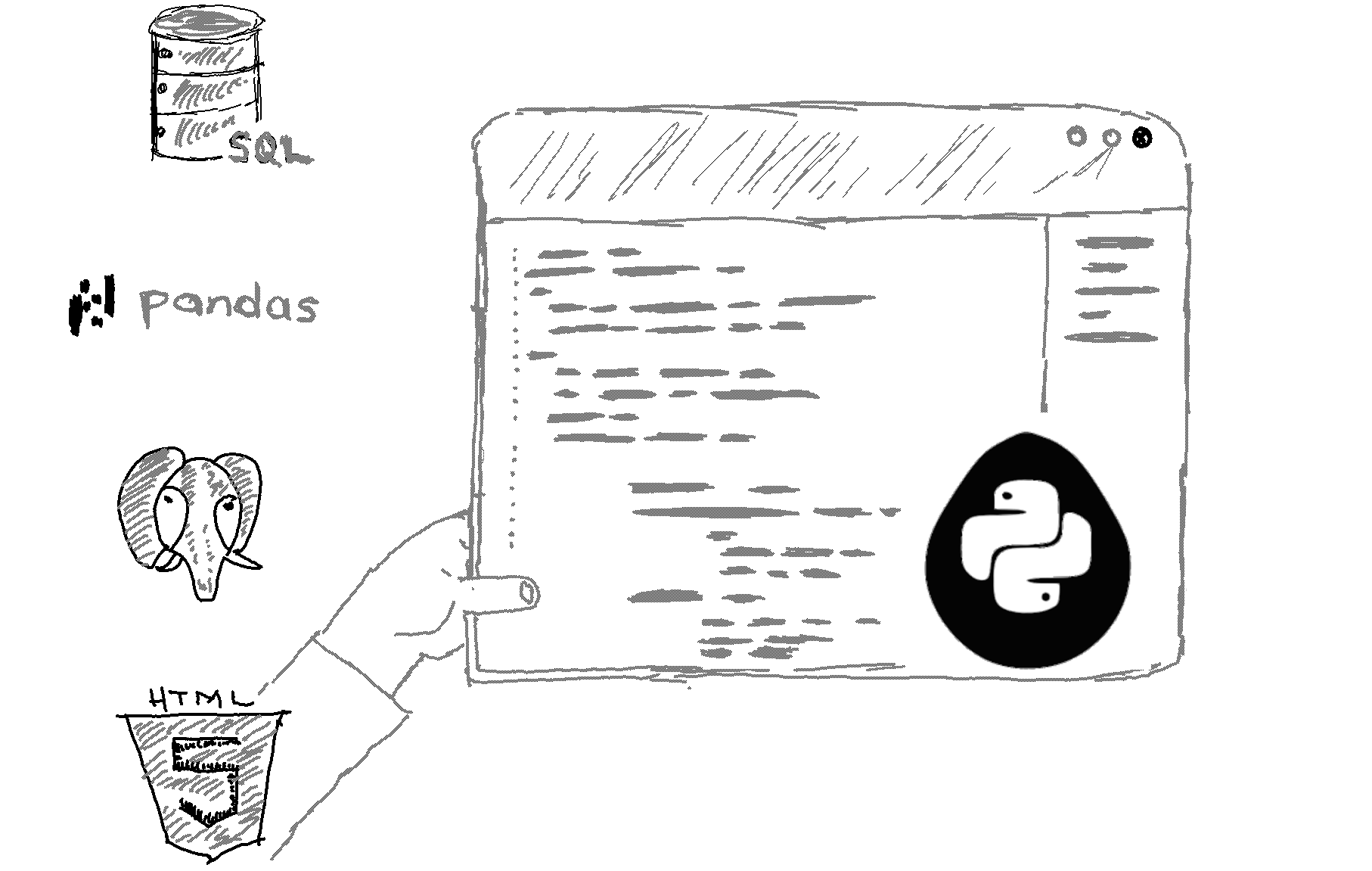# Here is how to parse a string to a float or integer in Python.

```# The string to be converted
num_string = "3.14159"

# Convert the string to a float
num = float(num_string)

# Print the first result
print(num_string)

# The string to be converted
num_string1 = "42"

# Convert the string to an int
num = int(num_string1)

# Print the second result
print(num_string1) ```

Output
```3.14159
42```

Explanation

To parse a string to a float or int in Python, you can use the float() and int() methods, respectively. These methods take a string as their argument and attempt to convert it to a float or int, respectively. If the conversion is successful, they return the converted value; otherwise, they raise a ValueError exception. Our first example would convert the string in the num_string variable to a float and store it in a variable called num. You can then use this variable in your code as needed.

Similarly, if you have a string that represents an integer, you can use the int() method to convert it to an int. Our code would convert the string in the num_string variable to an int and store it in a variable called num.

It's worth noting that the float() and int() methods can raise a ValueError exception if the string they are given cannot be converted to a float or int, respectively. For example, if you try to convert a string that contains letters or symbols other than digits and a decimal point (for float()) or only digits (for int()), a ValueError will be raised. In such cases, you should handle the exception in your code to prevent it from crashing.

Related HowTos
##### Recommended Course

Learn Flask development and learn to build cool apps with our premium Python course on Udemy.